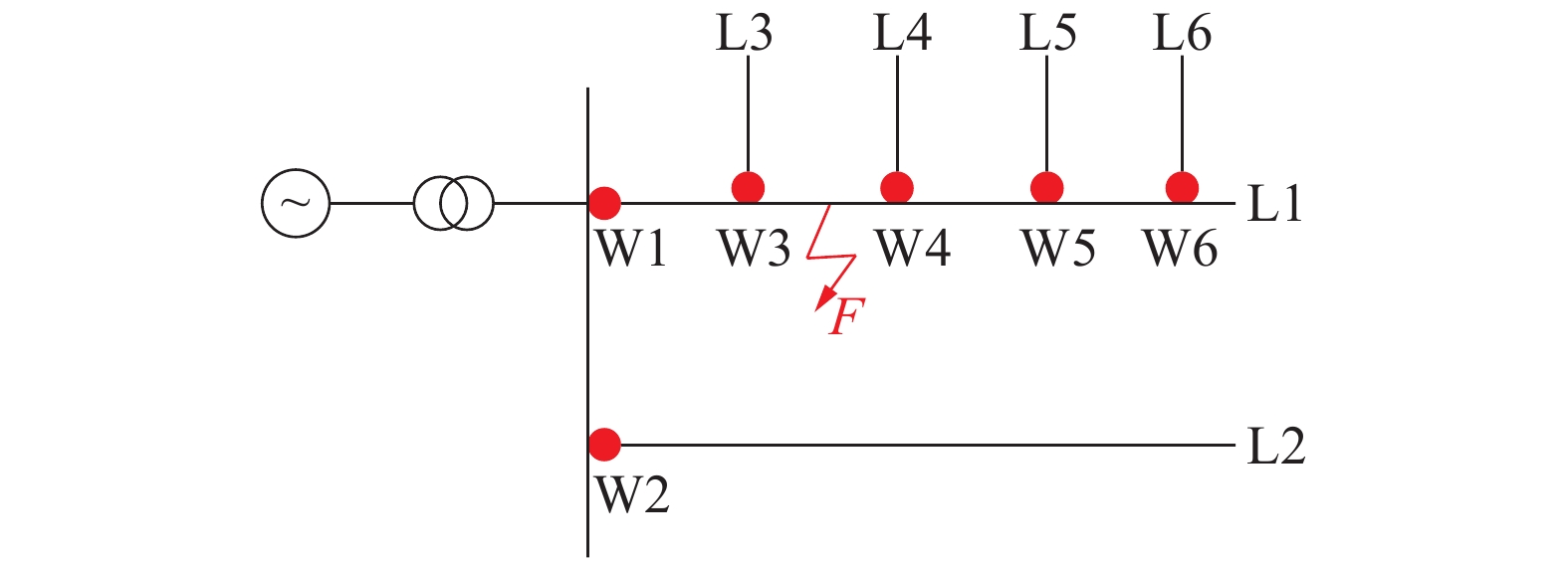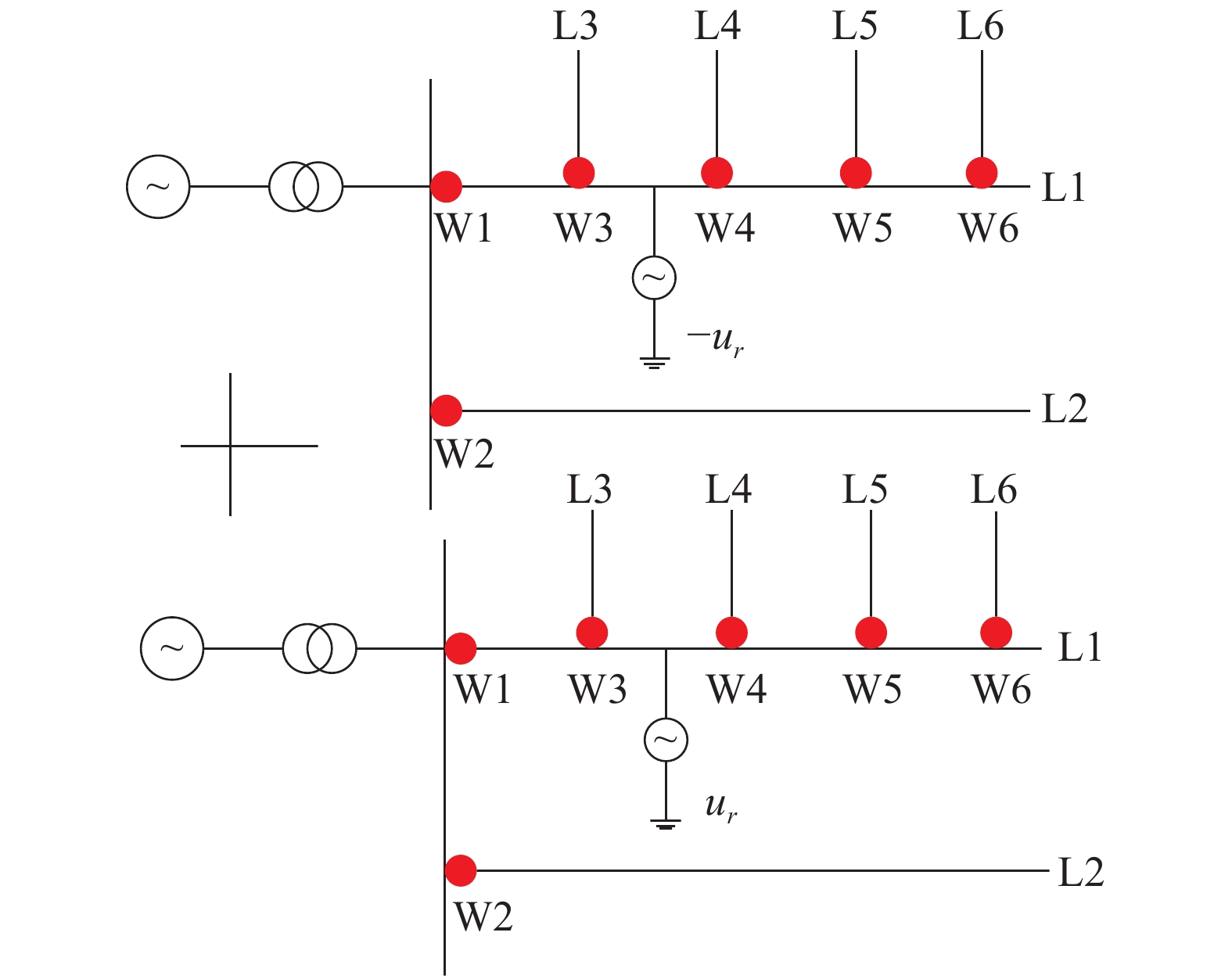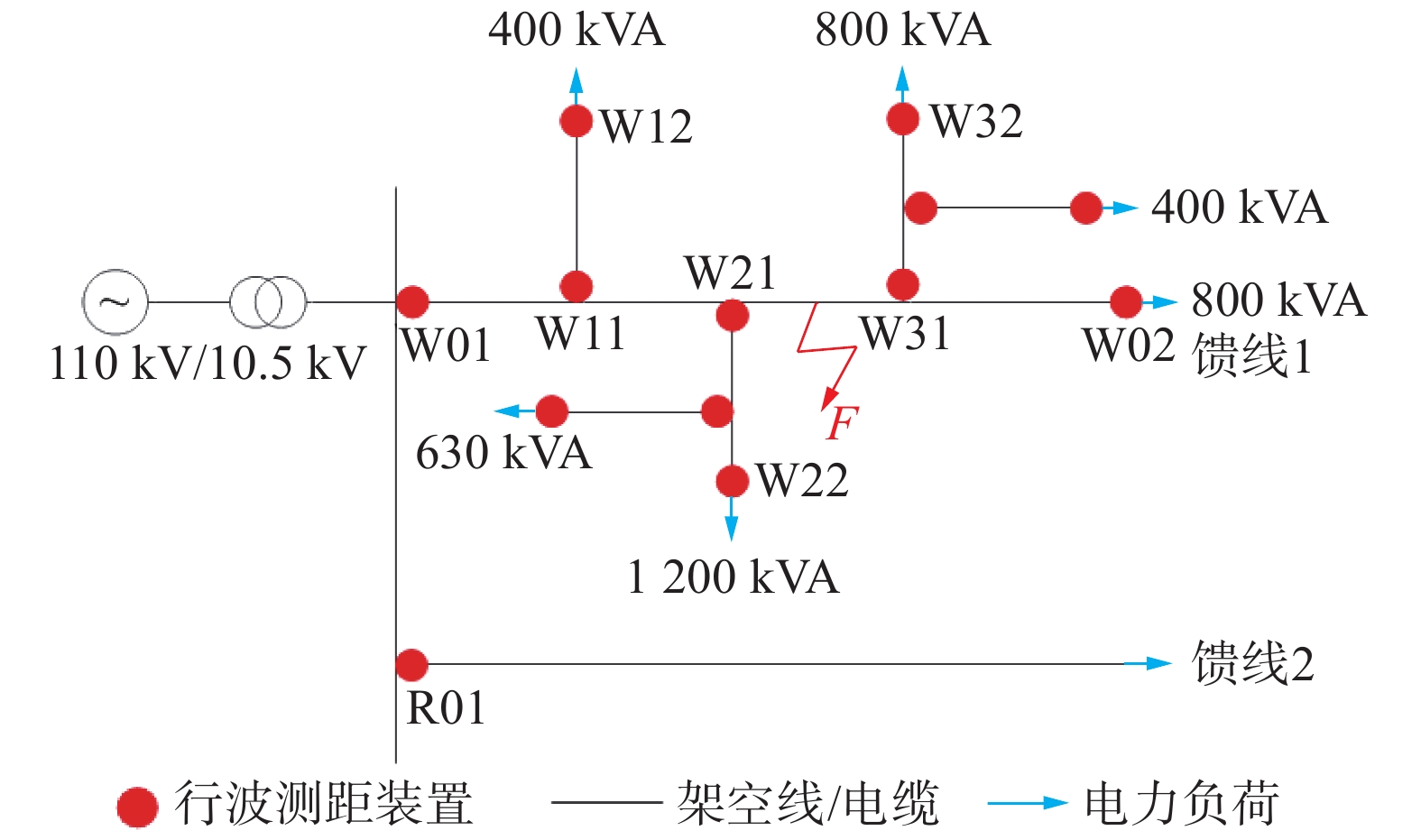﻿ 基于权重动态调整的树型配电网单相接地故障行波测距方法
«上一篇文章快速检索 高级检索

 应用科技2020, Vol. 47Issue (2): 53-58  DOI: 10.11991/yykj.2019070090

### 引用本文LYU Feng, CAI Chao, DING Jianzhong, et al. Traveling wave fault location method for single-phase grounding fault in tree-type distribution network based on dynamic weight adjustment[J]. Applied Science and Technology, 2020, 47(2): 53-58. DOI: 10.11991/yykj.201907009.### 文章历史

1. 国网江苏省电力有限公司无锡供电分公司，江苏 无锡 214061;
2. 河海大学 能源与电气学院，江苏 无锡 211100

Traveling wave fault location method for single-phase grounding fault in tree-type distribution network based on dynamic weight adjustment
LYU Feng1, CAI Chao2, DING Jianzhong1, YUAN Haixing1, SHEN Haiping1, HU Jinfeng1, SUN Guoqiang2
1. State Grid Jiangsu Power Supply Company Wuxi Branch, Wuxi 214061, China;
2. College of Energy and Electrical Engineering, Hohai University, Nanjing 211100, China
Abstract: Aiming at the problem that there are numerous branches in the tree-type distribution network and it is difficult to accurately select lines, the polarity relationship of initial current and voltage traveling waves of different lines is found through analysis in this paper, which improves the accuracy of branch line selection of distribution network. Because the zero-mode wave velocity in cable hybrid transmission line is not stable, it is difficult to obtain it precisely through line parameters. A traveling wave fault location method for single-phase grounding fault in tree-type distribution network is proposed based on dynamic weight adjustment. Based on the theory of two-terminal traveling wave fault location, the existing multi-terminal traveling wave fault location devices are combined freely, and the results of multi-group fault location are obtained. By comparing the errors of fault location results in history, the weight of each wave fault location device group is dynamically adjusted. Then, the fusion decision of fault information is made, and the final fault location is given. Therefore, the fault information of traveling wave fault location devices group on the same side of fault point is utilized in this paper to measure its wave velocity online, so that the wave velocity value used in fault location is more practical. Finally, PSCAD software is used to simulate and verify a practical 10 kV distribution system. The simulation results show that the proposed method is not affected by neutral grounding modes, and can realize accurate grounding fault location at different transition resistances and different operation modes of the system.
Keywords: weight adjustment    distribution network    single-phase grounding fault    traveling wave fault location    error compare    polarity relationship    fault line selection    traveling wave velocity

1 多分支配电网故障选线原理和方法

1.1 初始行波电压电流极性比较Download: 图 1 单相单电源多分支配电系统拓扑图Download: 图 2 单相单电源多分支配电系统故障后网络分解

 $\left\{ \begin{array}{l} {i_{{\rm{W}}1}} = - \displaystyle\frac{{{u_r}}}{{{Z_1}}}\\ {i_{{\rm{W}}2}} = \displaystyle\frac{{2{u_r}}}{{{Z_1} + {Z_2}}}\\ {i_{{\rm{W}}3}} = \displaystyle\frac{{2{u_r}}}{{{Z_1} + {Z_3}}}\\ {i_{{\rm{W}}4}} = \displaystyle\frac{{2{u_r}}}{{{Z_1} + {Z_4}}}\\ {i_{{\rm{W}}5}} = \displaystyle\frac{{2{u_r}}}{{{Z_1} + {Z_5}}} \end{array} \right., \left\{ \begin{array}{l} {u_{{\rm{W}}1}} = {u_r}\\ {u_{{\rm{W}}2}} = \displaystyle\frac{{2{Z_2}}}{{{Z_1} + {Z_2}}}{u_r}\\ {u_{{\rm{W}}3}} = \displaystyle\frac{{2{Z_3}}}{{{Z_1} + {Z_3}}}{u_r}\\ {u_{{\rm{W}}4}} = \displaystyle\frac{{2{Z_4}}}{{{Z_1} + {Z_4}}}{u_r}\\ {u_{{\rm{W}}5}} = \displaystyle\frac{{2{Z_5}}}{{{Z_1} + {Z_5}}}{u_r} \end{array} \right.$ (1)

 $\left\{ \begin{array}{l} {i_{{\rm{W}}1}} = - \displaystyle\frac{{2{u_r}}}{{{Z_3} + {Z_1}}}\\ {i_{{\rm{W}}2}} = \displaystyle\frac{{4{Z_1}{u_r}}}{{({Z_3} + {Z_1})({Z_1} + {Z_2})}}\\ {i_{{\rm{W}}3}} = - \displaystyle\frac{{{u_r}}}{{{Z_3}}}\\ {i_{{\rm{W}}4}} = \displaystyle\frac{{4{Z_1}{u_r}}}{{({Z_3} + {Z_1})({Z_1} + {Z_4})}}\\ {i_{{\rm{W}}5}} = \displaystyle\frac{{4{Z_1}{u_r}}}{{({Z_3} + {Z_1})({Z_1} + {Z_5})}} \end{array} \right., \left\{ \begin{array}{l} {u_{{\rm{W}}1}} = \displaystyle\frac{{2{Z_1}}}{{{Z_3} + {Z_1}}}{u_r}\\ {u_{{\rm{W}}2}} = \displaystyle\frac{{4{Z_1}{Z_2}}}{{({Z_3} + {Z_1})({Z_1} + {Z_2})}}{u_r}\\ {u_{{\rm{W}}3}} = {u_r}\\ {u_{{\rm{W}}4}} = \displaystyle\frac{{4{Z_1}{Z_4}}}{{({Z_3} + {Z_1})({Z_1} + {Z_4})}}{u_r}\\ {u_{{\rm{W}}5}} = \displaystyle\frac{{4{Z_1}{Z_5}}}{{({Z_3} + {Z_1})({Z_1} + {Z_5})}}{u_r} \end{array} \right.$ (2)

1.2 多分支配电网故障选线方法

 ${M_d} = {U_{{M_0}}} \times {I_{{M_0}}}$

2 缆线混联线路故障行波波速的确定

 $v = \frac{l}{{\left| {{t_1} - {t_2}} \right|}}$ (3)

3 行波测距装置组权重动态调整方法

 ${r_{{i_n}}} = ({p_i} + {q_i} \times \frac{1}{{{\delta _i}}}/\sum\limits_{j \in {N_j}} {1/{\delta _j}} )/({q_i} + {q_i})$ (4)

4 基于权重动态调整的配电网故障测距方法

1）采集故障初始电压电流行波信息，根据小波变换奇异性检测方法获取故障初始行波到达时刻；

2）根据故障初始电压电流行波极性比较，进行多分支配电线路的故障选线；

3）根据线路参数确定初始行波波速，利用故障所在线路两端行波测距装置进行故障点的预定位，从而将线路配置的行波测距装置分为故障点l侧装置与故障点r侧装置；

4）利用故障点同侧行波测距装置的初始行波到达时刻与两测距装置之间的距离，在线测量行波波速。

5）选取临近2次故障仿真或实测数据的误差比较，对当前权重值进行动态调整，建立权重修正表。

6）基于双端行波测距原理，计算各行波测距装置组的定位结果，结合其修正后的权重值，进行最终的融合决策，进而确定故障点位置；

7）将该次故障中各测距装置组的结果与真实故障点位置比较，根据其误差大小对其权重值进行调整，为下一次故障测距做准备。

5 仿真验证Download: 图 3 树型配电网故障仿真模型表 1 故障行波电压电流极性比较结果表 2 故障行波初始波头到达时刻表 3 架空线路故障电压行波波速表 4 各行波测量装置组的故障定位结果表 5 各测量装置组权重更新值表 6 行波测量装置组的融合决策结果

6 结论

1)分析各线路始端电压电流极性关系，解决了配电网多分支线路选线准确率低的问题；

2)充分利用多源量测冗余信息实现缆线混连线路行波波速的在线测量；

3)根据测距误差值动态调整各测距装置组的权重，从而进行融合决策，有效提高了配电网测距的可靠性和准确性。

  傅国庆. 暂态行波信息融合的输电线路故障定位方法研究[D]. 北京: 中国矿业大学, 2016. (0)  张帆, 潘贞存, 张慧芬, 等. 树型配电网单相接地故障行波测距新算法[J]. 中国电机工程学报, 2007, 27(28): 46-52. DOI:10.3321/j.issn:0258-8013.2007.28.009 (0)  季涛, 孙同景, 徐丙垠, 等. 配电混合线路双端行波故障测距技术[J]. 中国电机工程学报, 2006, 26(12): 89-94. DOI:10.3321/j.issn:0258-8013.2006.12.016 (0)  何晓, 雷勇, 周聪聪, 等. 消除零模波速影响的配电网单端行波故障测距算法[J]. 电力系统保护与控制, 2016, 44(23): 39-45. (0)  高杰, 程启明, 程尹曼, 等. 基于量子遗传双稳态系统的配电网故障选线方法[J]. 电力自动化设备, 2018, 38(5): 164-170, 203. (0)  曹明杰, 邹晓松, 袁旭峰, 等. 配电网接地故障测距改进方法研究[J]. 现代电子技术, 2019, 42(11): 117-120, 124. (0)  阙波, 陈蕾, 邵学俭. 一种配电网单相接地故障判断新方法及其应用[J]. 高压电器, 2017, 53(6): 191-196. (0)  王云鹤. 基于暂态分量的配电网故障定位[D]. 哈尔滨: 东北农业大学, 2016. (0)  张倩. 电力系统行波测距方法和发展[J]. 山东工业技术, 2019(3): 210-211. (0)  黄荣辉, 李勋, 张宏钊, 等. 线缆混合输电线路故障组合行波测距方法及影响因素研究[J]. 电力系统保护与控制, 2018, 46(5): 73-81. DOI:10.7667/PSPC170248 (0)  徐鹏, 杨胜春, 李峰, 等. 基于层次分析和变权重机制的电网安全指标计算及展示方法[J]. 电力系统自动化, 2015, 39(8): 133-140. DOI:10.7500/AEPS20140728014 (0)  牛海清, 郭少锋, 余佳. 复杂敷设输电电缆线路载流量瓶颈的时空特性[J]. 南方电网技术, 2018, 12(5): 44-50. (0)